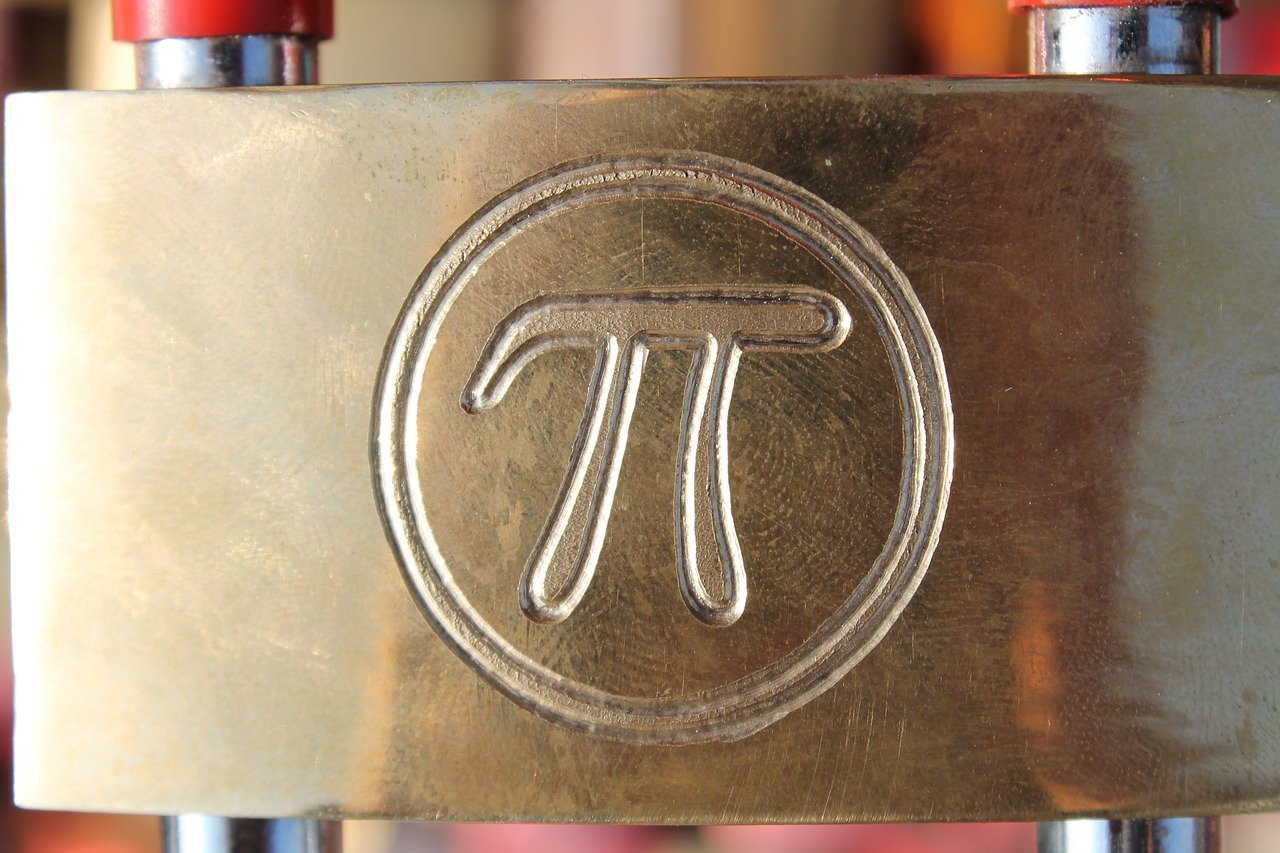# Origin of PI

Pi can be said as one of the mathematical calculations that are very well-known to human beings. Many civilizations had their own style of calculations for a particular apparent stream number which does not have any patterns on it.

The origin of pi was from the Egyptians and also they named it pi in 1650 BC with the calculation of (4/3) ^4 which is approximately 3.1604. Even the Babylonians calculated pi in a different mode which is (25/8) which is more than .50% of the normal pi value from the Egyptians.

Then the first calculation of pi in the fraction mode was done by the Greeks with the value of (22/7).

Then, since Persians are the greatest enemy of the Greeks, and found their pi value in a different way. That is (3*2) ^18 sides. With the help of this polygon, they found 2 pi is approximately 6.28318 where 1 pi is 3.14159.

The symbol of the pi is represented as ?. This symbol was first represented by William Jones, a Welsh mathematician during the year 1706. The symbol ? has become a most important symbol that is used in physics as well as in mathematical constants. Do even several formulas include? the symbol in engineering, science, etc. ? can be said as an irrational number where it can’t be expressed with fraction digits.

For example, if x/y are two fractional numbers where x and y are called integers. The decimal representation of the result has no end and it can be calculated with no end. It can also be said as a transcended number which means that the numbers with no sequence of expressions like square root, powers, sums, etc.

This ? was a late achievement in mathematics history and was found only during the 19th century. There were loads of efforts on determining the value of pi more accurately as well as acceptable criteria in all parts of the world.

Even in the United States of America, pi day is been celebrated on March 14th each year and they are been followed in all schools by celebrating with pi symbol dish plates as well as a decimal symbol expression appearing on it.

To make it more special, Kate Bush released an album related to? on November 7th, 2005 with the beginning word of “3.14” in the song. The album’s name is Aerial.